suivant: Bibliographie monter: A Bloch Constant for précédent: -holomorphy and quasiregularity

# Final remarks

The Picard theorem follows from Bloch's theorem in one variable. It is then interesting to ask whether the same is possible in the case of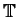-holomorphic mappings in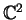. However, here we can directly find a Picard theorem without invoking our Bloch theorem.

Theorem 10 (Picard)   Let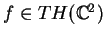. If there are two bicomplex numbers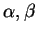such that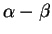is invertible and for which the set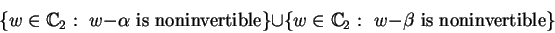is not in the range of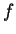, thenis constant.

Proof. We have just to apply the so-called little Picard theorem"  to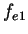and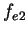. The fact thatis invertible will insure us thatwill be equal to a bicomplex number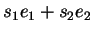with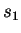and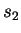nonzero. Let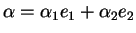,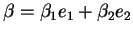. Supposetakes the value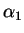at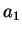. There exist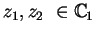such that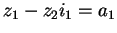. Thus,Contradiction. Hence,omits. Similarly,omits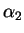,omits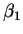andomits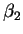. Since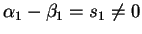,andare distinct. Similarly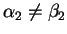.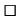In the same way, it is possible to find also a Casorati-Weierstrass theorem:

Theorem 11 (Casorati-Weierstrass)   Letwith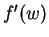not identically noninvertible. Then,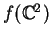is dense in.

Proof. The hypotheses imply that we can write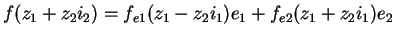with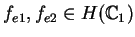and nonconstant. Then we can apply the Casorati-Weierstrass theorem for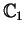toandin order to prove that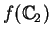is dense in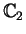.A famous example of Fatou and Bieberbach (see ) shows that the usual formulation of the Picard theorem in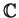does not extend to holomorphic mappings in.

In this connection, we have some interesting consequences of Theorem 11 which can be interpreted as an other kind of little Picard theorem for bicomplex numbers:

Corollary 1   There is no nondegenerate-holomorphic mapping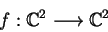such that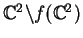contains a ball.

Corollary 2   Fatou-Bieberbach examples cannot be-holomorphic mappings, i.e. they connot satisfy the complexified Cauchy-Riemann equations.

For a beautiful formulation of Picard's theorem which holds in higher dimensions, see . Also, for a version of Picard's theorem for quasiregular mappings see Rickman .suivant: Bibliographie monter: A Bloch Constant for précédent: -holomorphy and quasiregularity
Dominic Rochon
2000-07-26